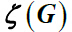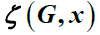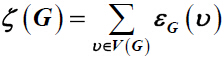# 图运算下的总离心率及多项式The Total Eccentricity and Polynomial of Some Graph Operations

DOI: 10.12677/AAM.2015.44048, PDF, HTML, XML, 下载: 1,773  浏览: 5,404  国家自然科学基金支持

Abstract: Let G be a simple connected graph. The total eccentricityand total eccentricity polynomialof a graph G are defined asand, wheredenotes the eccentricity of vertex ν  in G. In this paper, the total eccentricity and total eccentricity polynomial of double cover graph and extended double cover graph and subdivision graph of a given graph under the graph operations are computed and the exact expressions and some bounds are given.

  Wiener, H. (1947) Structural Determination of Paraffin Boiling Points. Journal of the American Chemical Society, 69, 17-20. http://dx.doi.org/10.1021/ja01193a005  Sharma, V., Gosami, R. and Madan, A.K. (1997) Eccentric Con-nectivity Index: A Novel Highly Discriminating Topological Descriptor for Structure-Property and Structure-Activity Studies. Journal of Chemical Information and Computer Science, 37, 273-282. http://dx.doi.org/10.1021/ci960049h  Morgan, M.J., Mukembi, S. and Swart, H.C. (2010) On the Eccentric Con-nectivity Index of a Graph. Discrete Mathematics, 311, 1229-1234.  Ilić, A. and Gutman, I. (2011) Eccentric Connectivity Index of Chemical Trees. Match Communications in Mathematical and in Computer Chemistry, 65, 731-744.  Bindusree, A.R., Lokesha, V. and P.S.R. (2015) Eccentric Connectivity Index and Polynomial of Some Graph Operations. British Journal of Mathematics & Computer Science, 6, 457-463.  Yarahmadi, Z., Moradi, S. and Došlić, T. (2014) Eccentric Connectivity Index of Graph with Subdivided Edges. Electronic Notes in Discrete Mathematics, 45, 167-176. http://dx.doi.org/10.1016/j.endm.2013.11.031